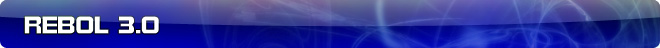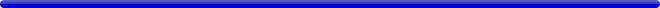# R3 Teaser: the STACK function

 Carl Sassenrath, CTO REBOL Technologies 2-Apr-2007 21:23 GMT Article #0075

Here is a debugging function that many REBOLers might find useful:

```USAGE:
STACK offset /block /word /func /args /size /depth /limit

DESCRIPTION:
Returns stack backtrace or other values.
STACK is a native value.

ARGUMENTS:
offset -- Relative backward offset (Type: integer!)

REFINEMENTS:
/block -- Block evaluation position
/word -- Function or object name, if known
/func -- Function value
/args -- Block of args (may be modified)
/size -- Current stack size (in value units)
/depth -- Stack depth (frames)
/limit -- Stack bounds (auto expanding)
```

(Currently, it's called stack, but it may be renamed because that word may be used in a number of scripts already.)

The offset is the number of stack frames to go back on the stack.

For, example, take this code:

```my-func: does [
loop 1 [
if true [
probe stack 0
print "there"
]
]
]
```

The zero offset indicates the top of the stack. When you run the code, you'll see:

```[stack if loop my-func]
```

That's a function invocation backtrace, with the most recent functions shown first.

I you only wanted to see functions prior to the stack call, you would use an offset of 1 and get:

```[if loop my-func]
```

### Other Information

The stack function can give you other useful information...

To get the code block where the function was called:

```>> stack/block 0
== [0 print "there"]
```

This is normally used by error functions to show where the error occurred. Notice that it does not show the function name. That's because functions don't strictly require names (can be lambda functions, that is unnamed) and functions can also be applied (with apply).

To get the name of a function in a stack (here it's just the stack function):

```>> stack/word 0
== stack
```

To get a function value from the stack:

```>> stack/func 0
== make native! [
{Returns stack backtrace or other values.}
offset [integer!] "Relative backward offset"
/block "Block evaluation position"
/word "Function or object name, if known"
/func "Function value"
/args "Block of args (may be modified)"
/size "Current stack size (in value units)"
/depth "Stack depth (frames)"
/limit "Stack bounds (auto expanding)"
]
```

That's a fully qualified function value, so you can use first, second, and even do it.

To find out the arguments to a function (including the refinements):

```>> stack/args 0
== [0 none none none true none none none none]
```

So, you can match that up with the function arguments block above.

To get the stack size (the number of values on the stack):

```>> stack/size 0
== 13
```

To get the stack depth (the number of functions or paths being evaluated):

```>> stack/depth 0
== 1
```

And finally, to get the stack bounds (note that this can change as the stack auto-expands):

```>> stack/limit 0
== 4096
```

So, there's a useful debug tool.

Better Name

As I noted, stack is probably not the final name. So, what would be the best name for such a function?